# Integer Data Types in C

## Integer Data Types in C Language with Examples

In this article, I will discuss Integer Data Types in C Language with examples. Please read our previous article discussing the basics of Data Types in C. We already discussed in our previous article that the Integer data type is divided into six classifications, as shown in the below image.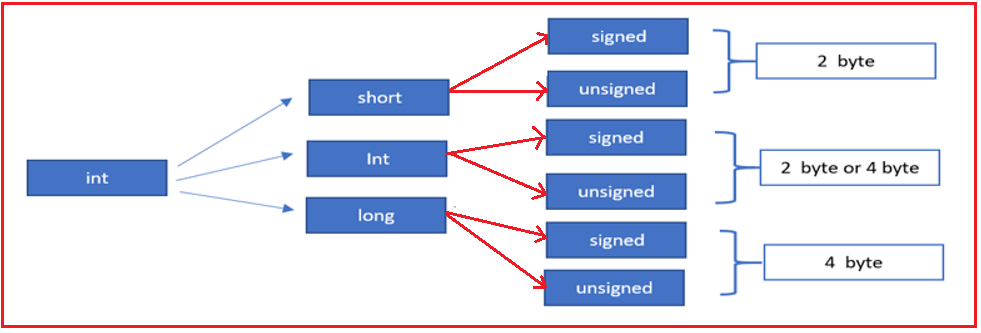The first classification is the short type. Short is again divided into two sub-classifications: signed short and unsigned short. Whether it is a signed short or an unsigned short, it occupies two bytes of memory.

###### What is a signed data type?

We can store positive and negative values using the signed data type.

###### What is the unsigned data type?

Using unsigned data types, we can store only positive values.

##### Using 2 bytes of memory, what minimum and maximum values can we store?

To understand this, look at the memory allocation process. Here, I am taking two bytes of memory. 1 byte equals 8 bits, so 2 bytes equals 16 bits. And it takes only binary values i.e. 0 and 1. Now, if we place zeros in all 16 places then the value will be zero, which is the minimum we can store in 2 bytes memory location, as shown in the below image.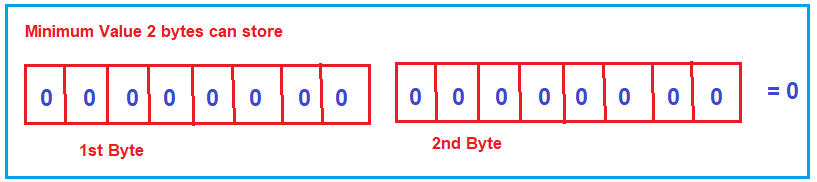If we place all ones in all 16 bits, the value is 65535. So, the maximum integer value we can store in 2 bytes is 65535, as shown in the below image.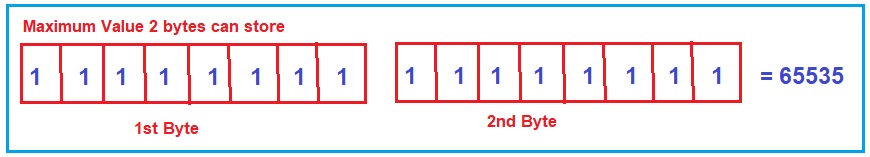So, using 2 bytes of memory, the minimum integer value we can store is 0, and the maximum integer value we can store is 65535. Now, let us come to the signed and unsigned data type. 1 byte is 8 bits, and 2 bytes is 16 bits. The unsigned short data type can store only positive integer values ranging from 0 to 65535, as shown in the below image.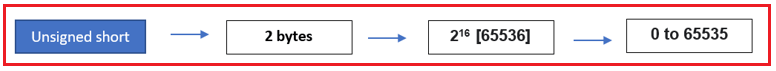The signed short data type can store both positive and negative values. So, divide the value by 2, i.e., 65536/2, which will result in 32768. The negative or minus value always starts from -1, -2, up to -32768. The positive value starts from 0, 1, and up to 32767 for signed shorts, as shown in the image below.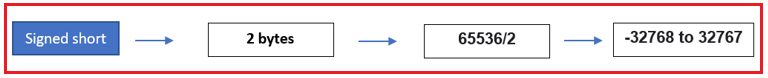##### Declaration of signed short Data Type in C Language:

If you do not specify whether the variable is a signed or unsigned variable, by default, that is a signed variable and can accept both positive and negative values. Following are the examples of declaring signed short variables in c language

short a;
short int a;
signed short a;
signed short int a;

The format specifier is %d for all four signed declarations. We can declare a signed variable in our C program in these many ways.

##### Declaration of unsigned short Data Type in C Language:

In unsigned declarations, we must specify explicitly that these are unsigned declarations. The two ways to declare unsigned short data types in c language follow.

unsigned short a;
unsigned short int a;

For these two unsigned declarations, the format specifier is %u. So, these are a total of six declarations about a short data type. First, four come under the signed short declarations, and the last two come under the unsigned declarations. For a better understanding, please have a look at the below image.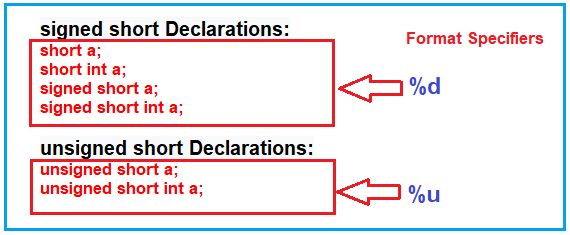###### The next important thing is what a format specifier is.

If you want to read the information or if you want to display the information, formatting is very important. In which format do you have to read the information, and in which format do you have to print the information? That you have to specify to the computer using a format specifier.

##### Example:

Now we will see some programs. I just want to print a small value on the console. We are writing the program, and the execution starts from the main method. I declare one variable, a short variable, and then print it on the console.

```#include <stdio.h>
#include <stdlib.h>
int main()
{
short a = 10;
system("cls");
printf("%d", a);
return 0;
}
```

Output: 10

In the above example, short a = 10; declaring a variable of type short and assigned with value 10. We want to print the value of the variable on the console, so here, we are using printf function, which belongs stdio.h header file. Inside the double quotes, we have to write the format specifier. As variable a is a signed variable, the format specifier is %d, and we want to print the value of a. The Value of a is 10, so the program output will be 10.

Note: To clear the screen in the Linux system, use system(“clear”) function, which is included in stdlib.h, and if you use this in the window, use the system(“cls”).

This is a very simple program, and here, the format specifier is very important. With the help of a format specifier only we are reading the elements and printing the elements.

##### Complex Examples using Short Data Type in C Language:

Next, we will see some complex programs. Consider the limits of signed short data type in the form of a circle. The range of minimum and maximum values of signed short data type is -32768 to +32767 as shown in the below image.

For any value you want to count, whether +VE values or -VE values, the count is always going to start from 0. Positive values will be counted in a clockwise direction, and the maximum value is 32767. The negative values count will be the anti-clockwise direction, and it will start from 0, -1, -2, up to -32768. For better understanding please have a look at the below diagram.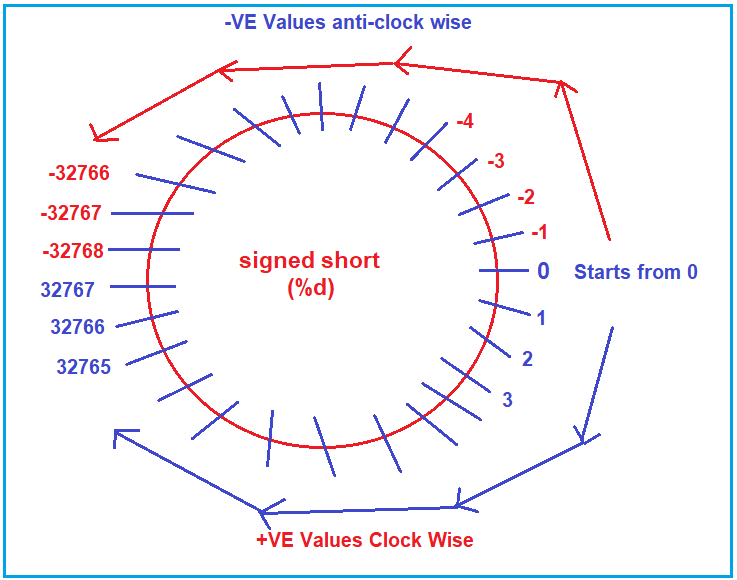Based on the above diagram, we will see one program. I just want to print a big value on the console. We are writing the program, and execution starts from the main method. I am declaring one short variable.

```#include <stdio.h>
int main()
{
short a = 32769;
printf("%d", a);
return 0;
}
```

Output: -32767

Why are we getting -32767, not 32769? As the value is a positive number the count will start clockwise from 0 and reach the maximum value of 32767. Now, please observe what the next value of 32767 in the clockwise direction; it is -32768 (32767+1 = 32768), and the next value is -32767 (32768+1 = 32769), and that is printed on the console. So, in this case, when the value exceeds, it will print a garbage value. In this case, it does not give any error; instead, it prints a garbage value.

##### Unsigned short Integer Data Type Example in C Language:

Now we will see one more program. Please have a look at the below example. Here, we declare a variable of unsigned short type but assign a negative value i.e. -5. We know that unsigned short only takes positive values. Let us first execute the program and see the output.

```#include <stdio.h>
int main()
{
unsigned short a = -5;
printf("%d", a);
return 0;
}
```

Output: 65531

To understand why we are getting 65531 in the output, we need to understand the unsigned short data type as a circle. The range of minimum and maximum values is 0 to 65535 for unsigned short, and it moves in a clockwise direction for +VE values and an anti-clockwise direction for -VE values, as shown in the image below.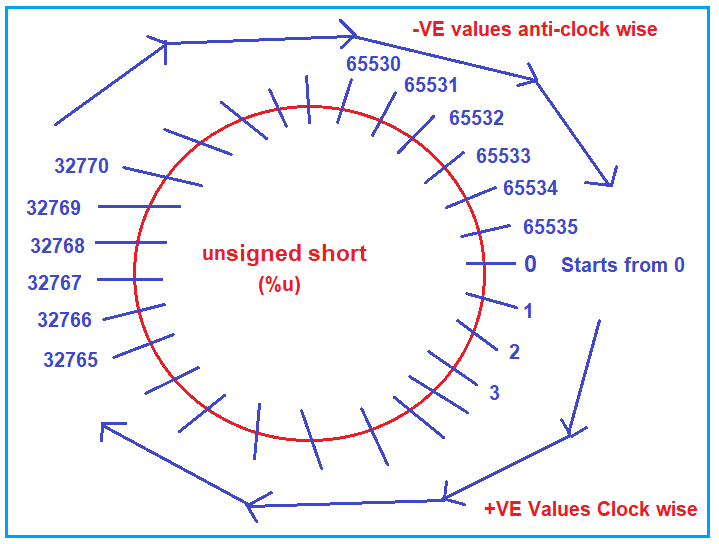Now, as we are assigning -5 to the unsigned variable, it will start counting in an anti-clockwise direction. So, it will start from 0, then 65535 for -1, 65534 for -2, 65533 for -3, 65532 for -4, and 65531 for -5, and it will store 65531 in the memory location, and that is what you can see in the memory output.

As we are using the %u format specifier, it will look into an unsigned circle diagram for the value of a. In the memory location, a value will be stored as 65531. Because -5 is not in the range of unsigned short variables, it will look in an anti-clockwise direction.

##### Example: unsigned short Integer in C Language

Now we will see one more program. In the program below, we declare an unsigned variable and assign it a value of 65538.

```#include <stdio.h>
int main()
{
unsigned short a = 65538;
printf("%u", a);
printf(" %d", a);
return 0;
}
```

Output: 2 2

Let us understand why we are getting 2 as the output. To understand this, first, we need to understand what value is going to be stored in the memory location for the variable a. So, here, the variable a data type is unsigned, so it will check the unsigned circle, which starts from 0 and ends with 65535 and counts the numbers in a clockwise direction. So, it will start from 0 to 65535 in the circle. What is the next value in the clockwise direction of 65535? It is 0. So, 0 for 65536, 1 for 65537, and 2 for 65538. So, in the memory location, it will store the value 2.

In the first printf statement, we have used the %u format specifier, so it will check the unsigned short circle and find value 2 is therein, and hence it will print that value. In the second printf statement, we have used the %d format specifier, so it will check the signed short circle and find value 2 is therein, and hence it will also print that value in the console.

Now we will see one more program. In the below program, we declare one unsigned short variable and assign it a value of -32772.

```#include <stdio.h>
int main()
{
unsigned short a = -32772;
printf("%u", a);
printf(" %d", a);
return 0;
}
```

Output: 32764 32764

Now it is your task to find out why we are getting 32764 as output.

##### Integer Data Types in C

In C programming, integer data types are used to store whole numbers (both positive and negative). The range of values that can be stored in these data types depends on their size and whether they are signed or unsigned. Here’s an overview:

###### int:
• The int data type is the most common integer type used in C.
• Its size is usually 4 bytes (32 bits), but this can vary based on the system architecture.
• The range is typically from -2,147,483,648 to 2,147,483,647 for a 32-bit system.
• Syntax: int a = 100;
###### short int or short:
• A smaller integer data type than int.
• Usually 2 bytes (16 bits) in size.
• The range for a signed short int is typically from -32,768 to 32,767.
• Syntax: short int b = 12345; or short b = 12345;
###### long int or long:
• Larger than int.
• Often 4 bytes (32 bits) are on a 32-bit system, and 8 bytes (64 bits) are on a 64-bit system.
• The range for a signed long int on a 64-bit system is typically from -9,223,372,036,854,775,808 to 9,223,372,036,854,775,807.
• Syntax: long int c = 123456789L; or long c = 123456789L;
###### long long int or long long:
• Even larger than long int.
• Typically, 8 bytes (64 bits) offer a wide range of values.
• The range is usually from -9,223,372,036,854,775,808 to 9,223,372,036,854,775,807.
• Syntax: long long int d = 123456789012345LL; or long long d = 123456789012345LL;
###### unsigned int, unsigned short int, unsigned long int, unsigned long long int:
• These are the unsigned variants of the respective integer types.
• They can only represent non-negative values, effectively doubling the upper limit of the range while having a lower limit of 0.
• For example, an unsigned int typically ranges from 0 to 4,294,967,295 on a 32-bit system.
• Syntax: unsigned int e = 4000000000U;

These integer types’ exact size and range can vary based on the system and compiler used. It’s always a good idea to use the sizeof operator to determine the size of a data type on a particular system. Additionally, the <stdint.h> header file in C99 and later standards provides fixed-width integers (like int16_t, int32_t, int64_t, and their unsigned variants) that have consistent sizes across different systems.

In the next article, I will discuss the Character Data Type in C Language with examples. In this article, I explain Integer Data Types in C Language with examples and I hope you enjoy this Integer Data Types in C Language article.